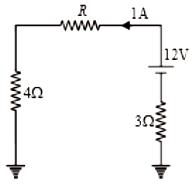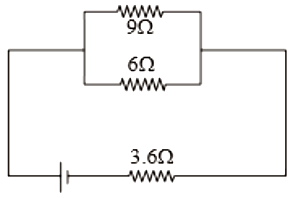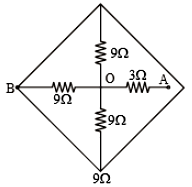For the circuit shown in the figure, the value of R must be1.  3$\mathrm{\Omega }$

2.  4$\mathrm{\Omega }$

3.  5$\mathrm{\Omega }$

4.  6$\mathrm{\Omega }$

Concept Questions :-

Kirchoff's voltage law
High Yielding Test Series + Question Bank - NEET 2020

Difficulty Level:

If I be the current limit of a fuse wire of length l and radius r, then select the appropriate relation

(1)

(2)

(3)

(4)

Concept Questions :-

Heating effect of current
High Yielding Test Series + Question Bank - NEET 2020

Difficulty Level:

1.  220

2.  440

3.  310

4.  550

Concept Questions :-

Derivation of Ohm's law
High Yielding Test Series + Question Bank - NEET 2020

Difficulty Level:

1.

2.

3.

4.   $4$

High Yielding Test Series + Question Bank - NEET 2020

Difficulty Level:

The sensitivity of a potentiometer can be increased by

2.  decreasing the length of potentiometer wire

3.  decreasing the current in the potentiometer wire

4.  all of these

Concept Questions :-

Meter bridge and potentiometer
High Yielding Test Series + Question Bank - NEET 2020

Difficulty Level:1.  36V

2.  18V

3.  9V

4.  Zero

Concept Questions :-

Heating effect of current
High Yielding Test Series + Question Bank - NEET 2020

Difficulty Level:

A current of 1 mA is flowing through a copper wire. How many electrons will pass a given point in one second

1.

2.

3.

4.

Concept Questions :-

Current and current density
High Yielding Test Series + Question Bank - NEET 2020

Difficulty Level:

The drift velocity of free electrons in a conductor is 'v' when a current 'i' is flowing in it. If both the radius and current are doubled, then drift velocity will be :

1.  v

2.

3.

4.  $\frac{\mathrm{v}}{8}$

Concept Questions :-

Current and current density
High Yielding Test Series + Question Bank - NEET 2020

Difficulty Level:

A battery has e.m.f. 4V and internal resistance r. When this battery is connected to an external resistance of 2 ohms, a current of 1 amp. flows in the circuit. How much current will flow if the terminals of the battery are connected directly

1.  1 Amp

2.  2 Amp

3.  4 Amp

4.  Infinite

Concept Questions :-

Emf and terminal voltage
High Yielding Test Series + Question Bank - NEET 2020

Difficulty Level:

The equivalent resistance between A and B is:1.

2.

3.

4.  $12\mathrm{\Omega }$

Concept Questions :-

Combination of resistors